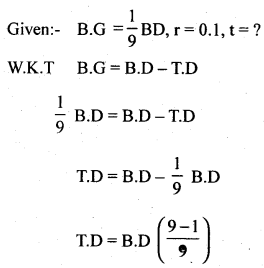# 2nd PUC Basic Maths Question Bank Chapter 8 Bill Discounting

Students can Download Basic Maths Question Bank Chapter 8 Bill Discounting Questions and Answers, Notes Pdf, 2nd PUC Basic Maths Question Bank with Answers helps you to revise the complete Karnataka State Board Syllabus and to clear all their doubts, score well in final exams.

## Karnataka 2nd PUC Basic Maths Question Bank Chapter 8 Bill Discounting

### 2nd PUC Basic Maths Bill Discounting Two Marks Questions and Answers

Question 1.
Define leagally due date? A bill was drawn on 14-3-2013 for 3 months, when does the bill fall leagally due?
L.D.D = Drawn date + Bill period + grace period of 3 daysQuestion 2.
Define true discount? and An bill drawn for 3 months was leagally due on 18-8-2012. Find the date of drawing of the bill.
Interest calculated on present value is called T.D.
L.D.D = D.D + B.P + 3 grace days
D.D = L.D.D – B.P – 3 grace daysQuestion 3.
Define Banker’s Discount? Find the Banker’s discount on Rs. 900 due 5 months hence at 16% p.a.
BD = Ftr ⇒ $$900 \times \frac{5}{12} \times \frac{16}{100}=60 \mathrm{Rs}$$Question 4.
Define Bankers gain and find the B.D on a bill of Rs 415 due 9 months hence at 15% p.a.
B.G = B.D -T.D                                                                                               .
B.D = Ftr
$$=415 \times \frac{9}{12} \times 0.15=\mathrm{Rs} .46 .68$$

Question 5.
Find the present value of Rs. 750 due 4 months hence at 15% p.a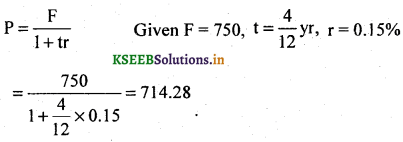Question 6.
True discount on a bill was Rs. 100 and Bankers gain was Rs. 10 what is the face value of the Bill?
GivenT.D= 100, B.G= 10,F = ?
BD -TD = BG ⇒ B.D = BG + TD = 100 +10 = 110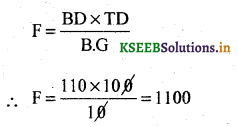Question 7.
A banker pays Rs. 2380 on a bill of Rs. 2,500 73days before the leagally due date. Find the rate of discount charged by the banker?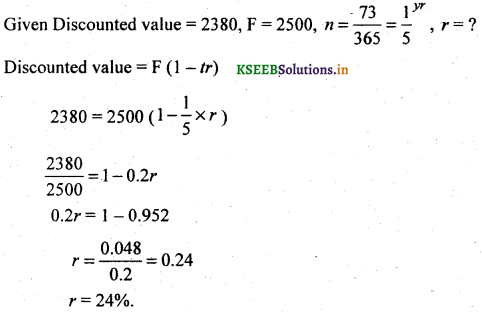Question 8.
The bankers discount and true discount on a sum of money due four months are respec­tively Rs. 510 and Rs. 500. Find the sum and the rate of interest
Given B.D = 510, TD = 500, t = months = $$\frac{1}{3}$$
B.G = B.D – T.D
= 510 – 500 -10 Rs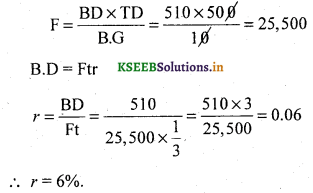Question 9.
BD and BG on a certain bill due after some time are Rs. 1250 and Rs. 50 respectively. Find the face value of the bill.
Given BD = 1250, B.G = 50, F = ?                               .
B.G =B.D – TD
∴ TD = B.D -B.G
= 1250 – 50
T.D = 1200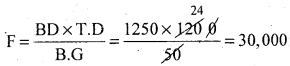Question 10.
A Banker pays Rs. 2340 on a bill of Rs. 2500,146 days before the leagally due date, what is the rate of discount charged by the banker.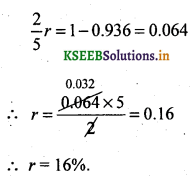Question 11.
The bankers gain on a certain bill due six months hence is Rs. 10, the rate of interest being 10% p.a. Find the face value of the bill.Question 12.
Find (i) The present worth and (ii) discounted cash value per Rs. 512.50 due 6 months at 15% p.a.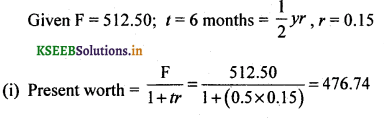(ii) Discount value = F (1 – tr)
= 512.50(1- 0.5 x 0.15)
= 512 x 0.925
= 474.06

### 2nd PUC Basic Maths Bill Discounting Three Marks Questions and Answers

Question 13.
The difference between B.D. and T.D. on a bill due after 6 months at 4% interest per annum is Rs. 20. Find T.D, B.D and Face value of the bill.Question 14.
Find Banker’s discount, True discount, True present value and Banker’s gain on a bill of Rs. 900 due 5 months hence at 16% p.a.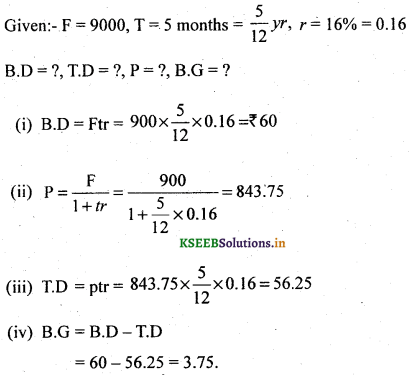Question 15.
A banker discounts a bill fr a certain amount having 73 days to run before it matures at 15% p.a. The discounted value of the bill is 970. What is the face value discounted value of the bill is 970. What is the face value of the bill? Also find, B.D.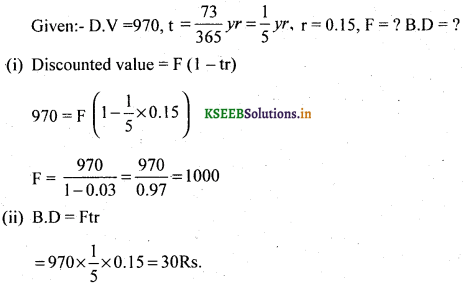Question 16.
A bill for Rs. 2,920 drawn at 6 months was discounted on 10-4-97 for Rs. 2916. If the discount rate is 5% p.a. on what date was the bill drawn?
F = 2920, D.V = 2916, r = 0.05,
Discounted date = 10 – 4 – 97
Discounted value = F(1 – tr)
2916 = 2920(1 – 0.05r)
$$\frac{2916}{2920}=1-0.05$$
0.05t = 1 – 0.99
0.05t = 0.01
$$t=\frac{0.01}{0.05} \times 365=73 \text { days }$$
Number of days from the leagally due-date to the discounted date is 73 days
10 – 4 – 97 + 73 days = 21 – 7 – 97
∴ Leagally due date is 21 – 6 – 97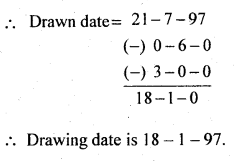Question 17.
A bill for Rs. 3500 due for 3 months wad drawn on 27th march 1996 and was discounted at the rate of 7% on 18th April, 1996. Find the B.D and the Discounted value of the bill.
Given:- F = 3500, Bill peiod = 3 months, D.D = 27 – 3 – 96
r = 0.07, B.D = ? D.V = ?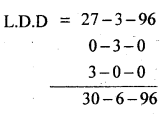∴ t = Number of days from April 18th to 30th June.
April + may + June
12 + 31 +30 = 73 days
$$t=\frac { 73 }{ 365 } =\frac { 1 }{ 5 } yr$$
(i) Discounted value = F(1 – tr)
$$=3500\left(1-\frac{1}{5} \times 0.07\right)$$
= 3451.00
∴ (ii) Bankers Discount = F – D.V
= 3500 – 3451
= 49Question 18.
A bill for Rs. 14,600 drawn at 3 months after date was discounted on 11 -11 – 95 for Rs.
14,320. It the discount rate is 20% p.a on what date was the bill drawn.
Given:- F = 14,600, D.V = 14,320 r = 0.2% t = ?
Discounted value = F (1 – tr)
14,320 = 14600(1 – 0.21)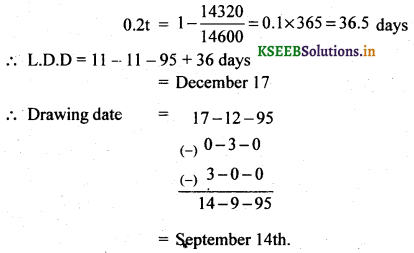Question 19.
A bill for Rs. 3,700 was drawn on 15-3-99 and made payable months after date. It was discounted on 6 – 4 – 99 at 16.5%. What was the (i) Bankers present value and (ii) How much has the banker gained on this transection.
Given:- F = 3700,D.D = 15 – 3 – 99, B.P = 3 months,
discounted on 6 – 4 – 99, R= 16.5% = 0.165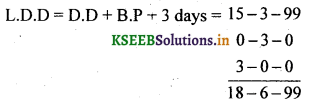t = No. of days from 6 – 4 – 99 to 18 – 6 – 99
April + May + June
24 + 31 + 18 = 73 days = $$\frac{1}{5} y r$$

(i) Banker’s present value = F (1 – tr)
= 3700(1- 0.2 x 0.165)
= 3577.9

(ii) B. D = Ftr = 3700 x 0.2x 0.165 = 122.10

(iii) $$\mathrm{T.D}=\frac{\mathrm{Ftr}}{1+t r}=\frac{122.10}{1+(0.2 \times 0.115)}$$=118.20
∴ B . G = B.D – T . D = 122.10 – 118.20 = 3.90Question 20.
The bankers gain on a bill is 1/9 th of the B.D, rate of interest being 10% p.a. Find the unexpired period of the bill.